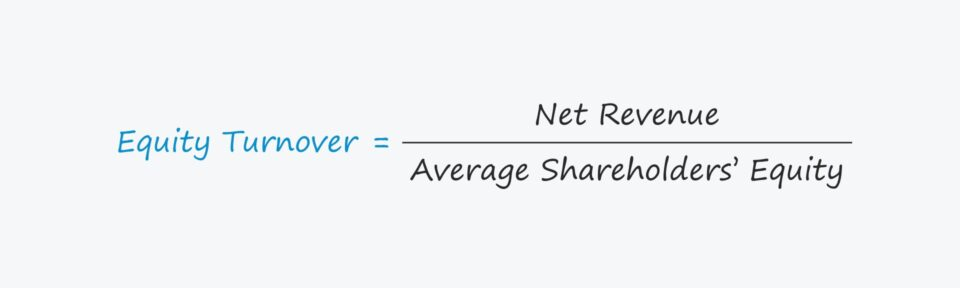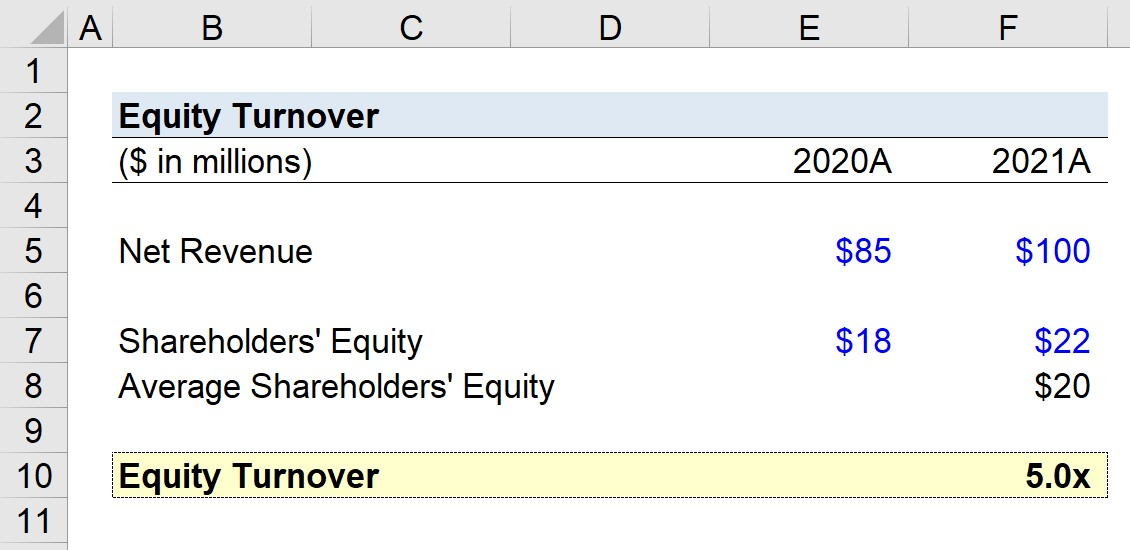# Equity Turnover Ratio

Guide to Understanding the Equity Turnover Concept## How to Calculate Equity Turnover

The equity turnover ratio, or “capital turnover”, measures the efficiency at which a company is utilizing its equity capital to generate revenue.

The ratio is computed by comparing a company’s net revenue to its average shareholders’ equity.

From the perspective of shareholders, the capital turnover metric is used to determine how well a company is using the capital contributed by equity holders.

If not, shareholders such as activist investors may try to actively pressure management to implement certain changes to fix the company’s operational issues (or sell their shares).

Calculating the turnover ratio requires two inputs.

1. Net Revenue → The net revenue figure adjusts a company’s gross revenue for any deductions related to customer returns, discounts, and allowances.
2. Average Shareholders’ Equity → The shareholders’ equity value is found on the balance sheet, so the amount referenced is the carrying balance recorded for book purposes as opposed to the market capitalization.

Usually, the capital turnover is calculated on an annual basis – i.e. full twelve-month period – to ensure seasonality is not skewing the metric.

Since the income statement covers a company’s financial performance across a specified time period whereas the balance sheet is a “snapshot” at a particular point in time, the average shareholders’ equity balance (between the starting and ending period) is used.

However, using the ending shareholders’ equity balance is still acceptable in most cases, as the difference in the resulting calculations is negligible.

## Equity Turnover Formula

The formula for calculating the equity turnover is as follows.

###### Formula
• Equity Turnover = Net Revenue ÷ Average Shareholders’ Equity

## How to Interpret the Capital Turnover Ratio

• “How much in revenue is generated per dollar of equity capital?”

If a company’s turnover is 2.0x, that means the company generates \$2.00 in revenue per \$1.00 of shareholders’ equity.

With that said, a higher capital turnover tends to be perceived more positively, as it implies more revenue creation per dollar of equity capital.

But the ratio is prone to manipulation and must take into consideration the specific context of the company under evaluation, such as the industry it operates within and the existing capital structure (i.e. debt-to-equity ratio).

In order for the capital turnover to be informative, the ratio must then be compared across the company’s historical performance as well as against its industry peers.

The benchmark target ratio varies substantially across different industries, making it crucial to only compare companies operating in similar sectors and with relatively similar capital structures.

## Equity Turnover Calculator – Excel Template

We’ll now move to a modeling exercise, which you can access by filling out the form below.Submitting ...

## Equity Turnover Example Calculation

Suppose we’re tasked with calculating the equity turnover of a company that generated \$85 million in 2020 and \$100 million in 2021.

• Net Revenue, 2020 = \$85 million
• Net Revenue, 2021 = \$100 million

As for the shareholders’ equity balances, the amount recorded for the fiscal year 2020 was \$18 million, followed by \$22 million in the year afterward.

• Shareholders’ Equity, 2020 = \$18 million
• Shareholders’ Equity, 2021 = \$22 million

The average shareholders’ equity between 2020 and 2021 is \$20 million.

• Average Shareholders’ Equity = (\$18 million + \$22 million) ÷ 2 = \$20 million

If we divide our hypothetical company’s net revenue in 2021 by our average shareholders’ equity, we arrive at an equity turnover of 5.0x.

• Equity Turnover = \$100 million ÷ \$20 million = 5.0x

The 5.0x equity turnover implies that for each \$1.00 of equity capital contributed by shareholders, \$5.00 is generated in net revenue.Step-by-Step Online Course

#### Everything You Need To Master Financial Modeling

Enroll in The Premium Package: Learn Financial Statement Modeling, DCF, M&A, LBO and Comps. The same training program used at top investment banks.